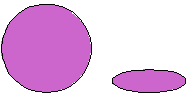Name: ___________________Date:___________________

 Email us to get an instant 20% discount on highly effective K-12 Math & English kwizNET Programs!

### MEAP Preparation - Grade 7 Mathematics1.145 Geometry Part Three - Review TestName: ___________________Date:___________________

### MEAP Preparation - Grade 7 Mathematics1.145 Geometry Part Three - Review Test

 Q 1: What is the area of a rectangle with length 5 inches and width 9 inches45 sq inches14 sq inches4 sq inches Q 2: Are these similar figures?NoYes Q 3: Two figures that are exactly the same shape and size are said to becongruentdifferentsimilar Q 4: How many square inches are there in a square foot. (hint: there are 12 inches in 1 foot)12 x 12 = 144 square inches12 + 12 = 24 square inches12 - 12 = 0 square inches Q 5: What is the volume of a cube with length 3 cm9 cubic cm27 cubic cm18 cubic cm Q 6: The two figures arenot congruentnot similar Q 7: What is the area of a rectangle whose length is 5 cm and width is 3 cm8 sq cm15 sq cm10 sq cm16 sq cm Q 8: The formula for volume of a figure with length L cm and width W cm and height H cm isV = L + W + HV = L x W x HV = L x W + H Question 9: This question is available to subscribers only! Question 10: This question is available to subscribers only!